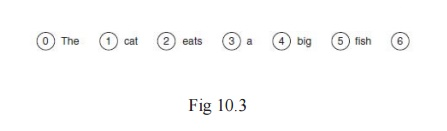Home | | Artificial Intelligence | Chart Parsing

# Chart Parsing

Parsing using transition networks is effective, but not the most efficient way to parse natural language. One problem can be seen in examining the following two sentences: 1. Have all the fish been fed? , Have all the fish.

Chart Parsing

Parsing using transition networks is effective, but not the most efficient way to parse natural language. One problem can be seen in examining the following two sentences: 1. Have all the fish been fed? , Have all the fish.

Clearly these are very different sentencesâ€”the first is a question, and the second is an instruction. In spite of this, the first threewords of each sentence are the same.

When a parser is examining one of these sentences, it is quite likely to have to backtrack to the beginning if it makes the wrong choice in the first case for the structure of the sentence. In longer sentences, this can be a much greater problem, particularly as it involves examining the same words more than once, without using the fact that the words have already been analyzed.Another method that is sometimes used for parsing natural language is chart parsing.

In the worst case, chart parsing will parse a sentence of n words in O(n3) time. In many cases it will perform better than this and will parse most sentences in O(n2) or even O(n) time.

In examining sentence 1 above, the chart parser would note that the words two children form a noun phrase. It would note this on its first pass through the sentence and would store this information in a chart, meaning it would not need to examine those words again on a subsequent pass, after backtracking.

The initial chart for the sentence The cat eats a big fish is shown in Fig 10.3 It shows the chart that the chart parse algorithm would start with for parsing the sentence.

The chart consists of seven vertices, which will become connected to each other by edges. The edges will show how the constituents of the sentence combine together.

The chart parser starts by adding the following edge to the chart:

[0, 0, Targetâ†’â€˘ Sentence]

This notation means that the edge connects vertex 0 to itself (the first two numbers in the square brackets show which vertices the edge connects).

Target is the target that we want to find, which is really just a placeholder to enable us to have an edge that requires us to find a whole sentence. The arrow indicates that in order to make what is on its left-hand side (Target) we need to find what is on its right-hand side (Sentence). The dot (â€˘) shows

what has been found already, on its left-hand side, and what is yet to be found, on its right-hand side. This is perhaps best explained by examining an example.

Consider the following edge, which is shown in the chart in Figure 10.4: [0, 2, Sentenceâ†’NounPhrase â€˘ VerbPhrase]

This means that an edge exists connecting nodes 0 and 2. The dot shows us that we have already found a NounPhrase (the cat) and that we are looking for a VerbPhrase.Once we have found the VerbPhrase, we will have what is on the left-hand side of the arrowâ€”that is, a Sentence.

The chart parser can add edges to the chart using the following three rules:

If we have an edge [x, y, A â†’ B â€˘ C], which needs to find a C, then an edge can be added that supplies that C (i.e., the edge [x, y, Câ†’ â€˘

E]), where E is some sequence of terminals or nonterminals which

can be replaced by a C).

If we have two edges, [x, y, A â†’ B â€˘ C D] and [y, z, C â†’ E â€˘}, then these two edges can be combined together to form a new edge:

[x, z, Aâ†’B C â€˘ D].

If we have an edge [x, y, A â†’ B â€˘ C], and the word at vertex y is of type C, then we have found a suitable word for this edge, and so we extend the edge along to the next vertex by adding the following edge: [y, y + 1, Aâ†’B C â€˘].

Study Material, Lecturing Notes, Assignment, Reference, Wiki description explanation, brief detail
Artificial Intelligence : Chart Parsing |